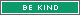1086 Posts
  Prev «  15 , 16 , 17 , 18 , 19  Next »   |

## Subject: Win 10 Geek Gold for Rolling the Geek Roller! (Roll 1947 to win)wizcreations wrote:
I plan to save \$ wizcreations previously rolled d2000 = (1183) = 1183 ( ) in the Steam sale.
That's impressive, especially since you'll probably buy 1d2000 = (643) = 643 games

EDIT: not actually that impressive
• [+] Dice rolls
• [+]
• 1479194. ColdFrog
• 1d2000 =
• (643) =
• 643
• Wed Jun 26, 2019 6:16 pm
I will go on a vacation for 1d2000 = (625) = 625 days
• [+] Dice rolls
• [+]
• 1479195. Elida
• 1d2000 =
• (625) =
• 625
• I will go on a vacation for
• Wed Jun 26, 2019 6:18 pm
d2000 = (566) = 566
• [+] Dice rolls
• [+]
• 1479436. CrazyCatman
• d2000 =
• (566) =
• 566
• Thu Jun 27, 2019 9:10 am
1d2000 = (1054) = 1054
• [+] Dice rolls
• [+]
• 1479448. Jrtolf
• 1d2000 =
• (1054) =
• 1054
• Thu Jun 27, 2019 11:23 am
d2000 = (1024) = 1024
• [+] Dice rolls
• [+]
• 1479465. Jlerpy
• d2000 =
• (1024) =
• 1024
• Thu Jun 27, 2019 12:40 pm
d2000 = (1544) = 1544
• [+] Dice rolls
• [+]
• 1479576. bulldog93
• d2000 =
• (1544) =
• 1544
• Thu Jun 27, 2019 3:57 pm
d2000 = (113) = 113
• [+] Dice rolls
• [+]
• 1479640. GeoffreyB
• d2000 =
• (113) =
• 113
• Thu Jun 27, 2019 5:10 pm
1d2000 = (71) = 71
• [+] Dice rolls
• [+]
• 1479938. ghostpants
• 1d2000 =
• (71) =
• 71
• Fri Jun 28, 2019 4:14 am
bleh d2000 = (399) = 399
• [+] Dice rolls
• [+]
• 1479943. wizcreations
• d2000 =
• (399) =
• 399
• bleh
• Fri Jun 28, 2019 4:49 am
When I finally finish a full run of Slay the Spire with the Ironclad, I'll score 1d2000 = (236) = 236 points
• [+] Dice rolls
• [+]
• 1480113. ColdFrog
• 1d2000 =
• (236) =
• 236
• Fri Jun 28, 2019 3:52 pm
d2000 = (252) = 252
• [+] Dice rolls
• [+]
• 1480134. bulldog93
• d2000 =
• (252) =
• 252
• Fri Jun 28, 2019 4:47 pm
d2000 = (709) = 709
• [+] Dice rolls
• [+]
• 1480172. GeoffreyB
• d2000 =
• (709) =
• 709
• Fri Jun 28, 2019 5:50 pm
1d2000 = (1298) = 1298
• [+] Dice rolls
• [+]
• 1480185. Jrtolf
• 1d2000 =
• (1298) =
• 1298
• Fri Jun 28, 2019 6:24 pm
when I lose a bet it will not be for 1d2000 = (990) = 990
• [+] Dice rolls
• [+]
• 1480267. AkamsB
• 1d2000 =
• (990) =
• 990
• Fri Jun 28, 2019 9:19 pm
I rolled an actual d2000 and got a D2000 = (515) = 515
• [+] Dice rolls
• [+]
• 1480334. wizcreations
• D2000 =
• (515) =
• 515
• Sat Jun 29, 2019 3:00 am
I virtually rolled a figurative d2000 and got a 1d2000 = (1883) = 1883
• [+] Dice rolls
• [+]
• 1480349. Jlerpy
• 1d2000 =
• (1883) =
• 1883
• Sat Jun 29, 2019 7:53 am
Rose
United States
New York City
NY
"I do what I do because it’s right! Because it’s decent! And above all, it’s kind! It’s just that… Just kind." -Dr. Who"And forget not that the earth delights to feel your bare feet and the winds long to play with your hair." - Khalil Gibran
1d2000 = (297) = 297
• [+] Dice rolls
• [+]
• 1480407. anjulka
• 1d2000 =
• (297) =
• 297
• Sat Jun 29, 2019 1:55 pm
1d2000 = (150) = 150
• [+] Dice rolls
• [+]
• 1480410. Jrtolf
• 1d2000 =
• (150) =
• 150
• Sat Jun 29, 2019 2:04 pm
A curve of all the rolled values is skewed d2000 = (756) = 756 left from center.
• [+] Dice rolls
• [+]
• 1480424. wizcreations
• d2000 =
• (756) =
• 756
• Sat Jun 29, 2019 3:18 pm
d2000 = (435) = 435
• [+] Dice rolls
• [+]
• 1480431. bulldog93
• d2000 =
• (435) =
• 435
• Sat Jun 29, 2019 3:52 pm
I lost 1d2000 = (1937) = 1937 pounds.
• [+] Dice rolls
• [+]
• 1480480. ghostpants
• 1d2000 =
• (1937) =
• 1937
• I lost
• Sat Jun 29, 2019 6:21 pm
d2000 = (1147) = 1147
• [+] Dice rolls
• [+]
• 1480482. GeoffreyB
• d2000 =
• (1147) =
• 1147
• Sat Jun 29, 2019 6:30 pm
d2000 = (1831) = 1831
• [+] Dice rolls
• [+]
• 1480555. CrazyCatman
• d2000 =
• (1831) =
• 1831
• Sun Jun 30, 2019 4:53 am
1d2000 = (1683) = 1683
• [+] Dice rolls
• [+]
• 1480563. ghostpants
• 1d2000 =
• (1683) =
• 1683
• Sun Jun 30, 2019 6:25 am
1d2000 = (1521) = 1521
• [+] Dice rolls
• [+]
• 1480618. Jrtolf
• 1d2000 =
• (1521) =
• 1521
• Sun Jun 30, 2019 11:53 am
  Prev «  15 , 16 , 17 , 18 , 19  Next »   |